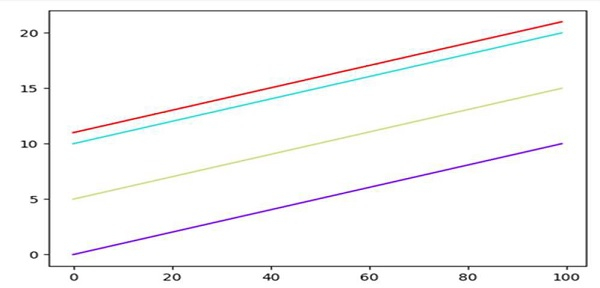# Using Colormaps to set the color of line in Matplotlib

First, we can create a list of colors, and then, we can use the colors while plotting a line in a loop.

## Steps

• Return evenly spaced numbers over a specified interval, store in x.

• Update x for four lines and get another variable for evenly_spaced_interval.

• Make a list of colors.

• Iterate color and set color for all the lines.

• To show the figure, use plt.show() method.

## Example

from matplotlib import pyplot as plt, cm
import numpy as np

x = np.linspace(0, 10, 100)
lines = [x, x+10, x+5, x+11]

evenly_spaced_interval = np.linspace(0, 1, len(lines))
colors = [cm.rainbow(x) for x in evenly_spaced_interval]
for i, color in enumerate(colors):
plt.plot(lines[i], color=color)

plt.show()

## Output